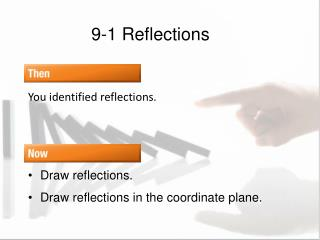DownloadDownload Presentation9-1 Reflections

# 9-1 Reflections

Download Presentation## 9-1 Reflections

- - - - - - - - - - - - - - - - - - - - - - - - - - - E N D - - - - - - - - - - - - - - - - - - - - - - - - - - -
##### Presentation Transcript

1. 9-1 Reflections You identified reflections. • Draw reflections. • Draw reflections in the coordinate plane.

2. Reflection She saw her reflection in the mirror. The trees were reflected in the lake. The sphere was so clear, I saw my reflection.

3. Definition of Reflection A reflection is a flipping of a figure over a line. This is the line of reflection. A reflection is a special type of transformation. Line of Reflection

4. Pre-Image/Image Pre-image the original figure Image the figure after a transformation A B B′ A′ C C′ l Pre-image (1) Image (2)

5. Pre-image/Image To tell the two images apart, use prime notation A′. When naming images of figures, list corresponding points in the same order.

6. Reflective Detective Fold a sheet of paper in half. Poke the tip of a pencil through the folded paper at 3 points that are not collinear. Open the paper and draw segments connecting the holes on each side of the fold. (You should have 2 triangles with the fold as the line of reflection.) Label all the points. Draw segments connecting the points of the image with corresponding points of the pre-image. Measure the distance from the vertices to the fold. Measure the angles these segments make with the fold.

7. Vocabulary Equidistant two points are the same distance from another point, segment or line. Bisector a figure is cut into two congruent halves. Perpendicular bisector a line or segment that divides the segment into two congruent segments and is perpendicular to it. A B l is a perpendicular bisector of AB l

8. p. 623

9. Step 2Locate W', X', Y', and Z' so that line p is the perpendicular bisector of Points W', X', Y', and Z' are the respective images of W, X, Y, and Z. Reflect a Figure in a Line Draw the reflected image of quadrilateral WXYZ in line p. Step 1Draw segments perpendicular to line p from each point W, X, Y, and Z.

10. Step 3Connect vertices W', X', Y', and Z'. Answer:Since points W', X', Y', and Z' are the images of points W, X, Y, and Z under reflection in line p, then quadrilateral W'X'Y'Z' is the reflection of quadrilateral WXYZ in line p.

11. A. B. C. D. Draw the reflected image of quadrilateral ABCD in line n.

12. Reflect a Figure in a Horizontal or Vertical Line A. Quadrilateral JKLM has vertices J(2, 3), K(3, 2), L(2, –1), and M(0, 1). Graph JKLM and its image over x = 1.

13. Use the horizontal grid lines to find a corresponding point for each vertex so that each vertex and its image are equidistant from the line x = 1. Answer:

14. Reflect a Figure in a Horizontal or Vertical Line Use the horizontal grid lines to find a corresponding point for each vertex so that each vertex and its image are equidistant from the line x = 1. Answer:

15. Reflect a Figure in a Horizontal or Vertical Line B. Quadrilateral JKLM has vertices J(2, 3), K(3, 2), L(2, –1), and M(0, 1). Graph JKLM and its image over y = –2.

16. Use the vertical grid lines to find a corresponding point for each vertex so that each vertex and its image are equidistant from the line y = –2. Answer:

17. A.B. C.D. A. Quadrilateral ABCD has vertices A(1, 2), B(0, 1), C(1, –2), and D(3, 0). Graph ABCD and its image over x = 2.

18. p. 625

19. Reflect a Figure in the x- or y-axis A. Graph quadrilateral ABCD with vertices A(1, 1), B(3, 2), C(4, –1), and D(2, –3) and its image reflected in the x-axis. Multiply the y-coordinate of each vertex by –1. (x, y)→ (x, –y) A(1, 1)→ A'(1, –1)B(3, 2)→ B'(3, –2)C(4, –1)→ C'(4, 1)D(2, –3)→ D'(2, 3) Answer:

20. Reflect a Figure in the x- or y-axis B. Graph quadrilateral ABCD with vertices A(1, 1), B(3, 2), C(4, –1), and D(2, –3) and its reflected image in the y-axis. Multiply the x-coordinate of each vertex by –1. (x, y)→ (–x, y) A(1, 1)→ A'(–1, 1)B(3, 2)→ B'(–3, 2)C(4, –1)→ C'(–4, –1)D(2, –3)→ D'(–2, –3) Answer:

21. A. Graph quadrilateral LMNO with vertices L(3, 1), M(5, 2), N(6, –1), and O(4, –3) and its reflected image in the x-axis. Select the correct coordinates for the new quadrilateral L'M'N'O'. A.L'(3, –1), M'(5, –2), N'(6, 1), O'(4, 3) B.L'(–3, 1), M'(–5, 2), N'(–6, –1), O'(–4, –3) C.L'(–3, –1), M'(–5, –2), N'(–6, 1), O'(–4, 3) D.L'(1, 3), M'(2, 5), N'(–1, 6), O'(–3, 4)

22. p. 626

23. Reflect a Figure in the Line y = x Quadrilateral ABCD with vertices A(1, 1), B(3, 2), C(4, –1), and D(2, –3). Graph ABCDand its image under reflection of the line y = x. Interchange the x- and y-coordinates of each vertex. (x, y)→ (y, x) A(1, 1)→ A'(1, 1)B(3, 2)→ B'(2, 3)C(4, –1)→ C'(–1, 4)D(2, –3)→ D'(–3, 2) Answer:

24. p. 626

25. 9-1 Assignment p. 627, 10-14 even, 20, 21, 26-27